# Buoyancy Dr Joel Cahoon Civil Engineering Summer 2009

• Slides: 37
Download presentationBuoyancy • • • Dr. Joel Cahoon Civil Engineering Summer 2009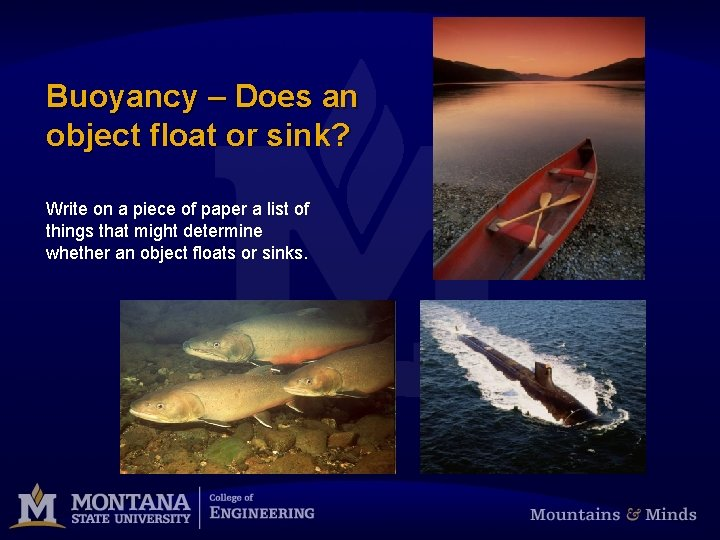Buoyancy – Does an object float or sink? Write on a piece of paper a list of things that might determine whether an object floats or sinks.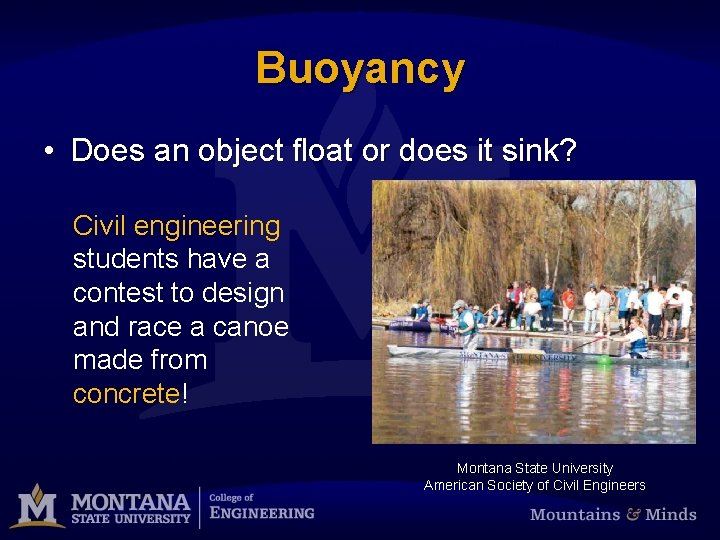Buoyancy • Does an object float or does it sink? Civil engineering students have a contest to design and race a canoe made from concrete! Montana State University American Society of Civil EngineersSome concrete canoes float better than others! University of Kansas – Daily Kansan Photo by Anna FaltermeierWhat is a Force? The weight of an object is a force. We will work with forces using the units of “pounds”. Pounds is abbreviated “lb”.Force This concrete canoe weighs 330 pounds. Miss St University Relations News Bureau (662) 325 -3442 Contact: Robbie Ward June 11, 2007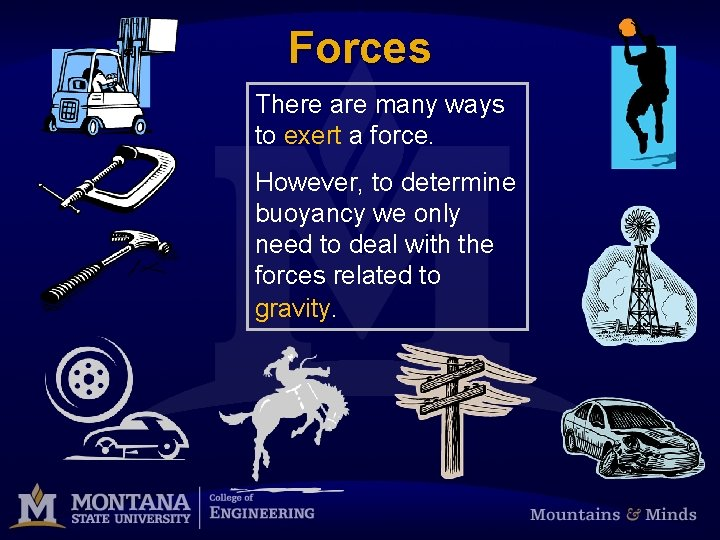Forces There are many ways to exert a force. However, to determine buoyancy we only need to deal with the forces related to gravity.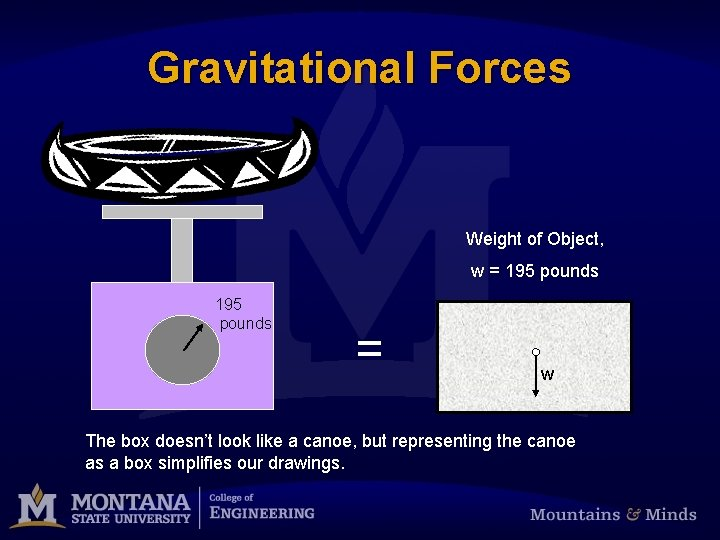Gravitational Forces Weight of Object, w = 195 pounds = w The box doesn’t look like a canoe, but representing the canoe as a box simplifies our drawings.What is a Force Balance? w Weight of Object, Fe w = 195 lb w + 195 lb = w Supporting Force, Fe = 195 lb The scale itself is providing the supporting force, and the supporting force is equal to the weight of the object. Note: Up is (+) Down is (-)Other Supporting Forces? The supporting force is from above. There is no supporting force from below. Supporting Force, Fe = 150 lb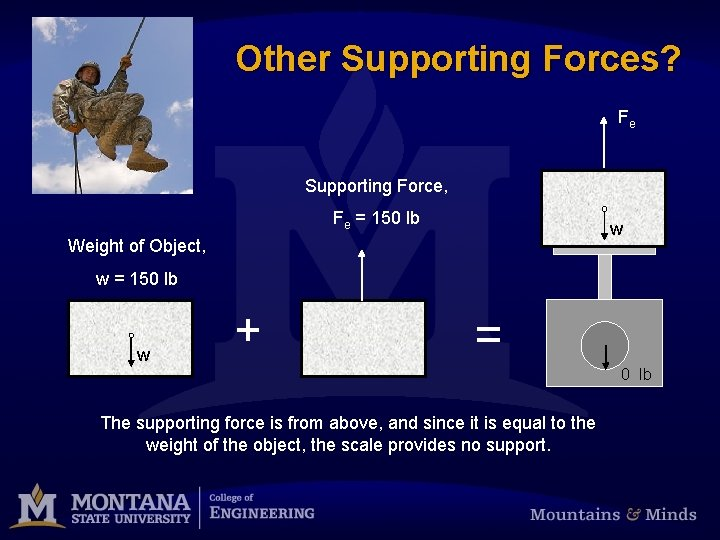Other Supporting Forces? Fe Supporting Force, Fe = 150 lb w Weight of Object, w = 150 lb w + = 0 lb The supporting force is from above, and since it is equal to the weight of the object, the scale provides no support.More Than 1 Supporting Force? Crouch on a bathroom scale. Have two strong friends start to lift you by your elbows. Is there a point where half your weight is supported by your friends and half your weight is supported by the scale? In the diagram, the block represents you. Fe = 0. 5 w w 0. 5 w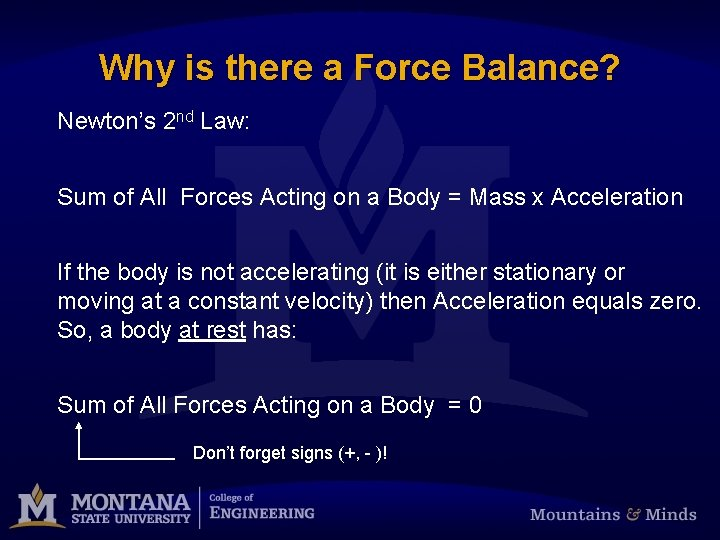Why is there a Force Balance? Newton’s 2 nd Law: Sum of All Forces Acting on a Body = Mass x Acceleration If the body is not accelerating (it is either stationary or moving at a constant velocity) then Acceleration equals zero. So, a body at rest has: Sum of All Forces Acting on a Body = 0 Don’t forget signs (+, - )!Force Balance on a Submerged Object Water Surface Supporting Force, Fe Weight of Object, w Buoyant Force, Fb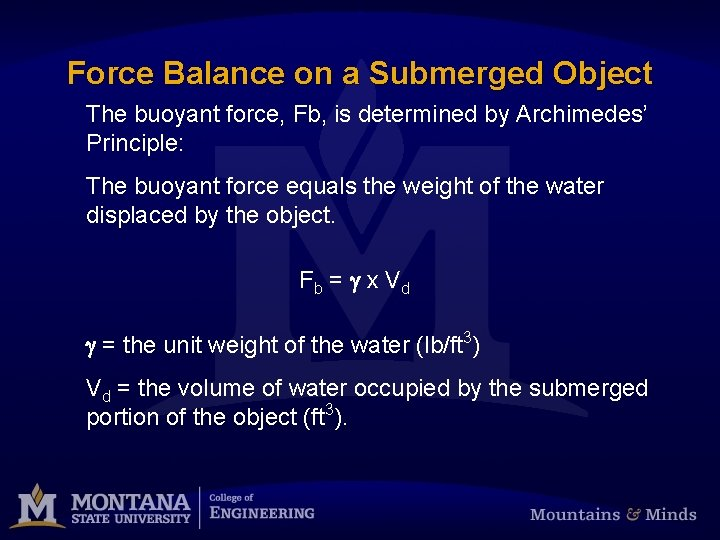Force Balance on a Submerged Object The buoyant force, Fb, is determined by Archimedes’ Principle: The buoyant force equals the weight of the water displaced by the object. F b = g x Vd g = the unit weight of the water (lb/ft 3) Vd = the volume of water occupied by the submerged portion of the object (ft 3).What is the Unit Weight of Water? A 1 foot cube of water weighs 62. 4 lb. So, for water: g = 62. 4 lb/1 cubic foot = 62. 4 lb/ft 3 Water 1 ft = 62. 4 lb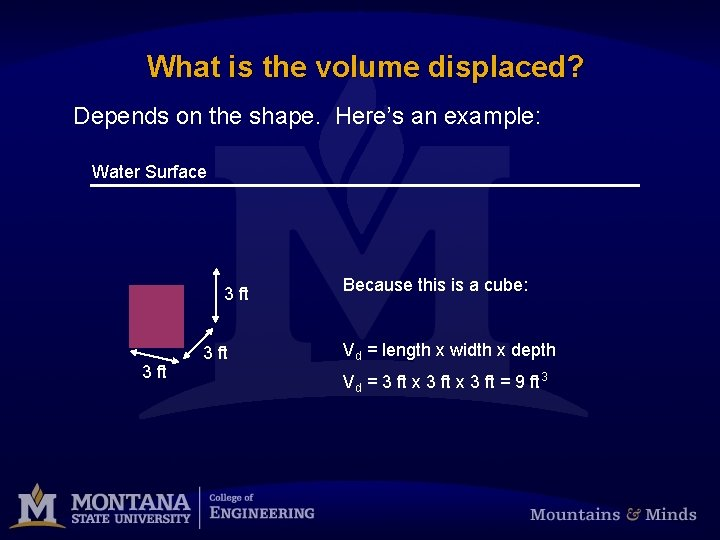What is the volume displaced? Depends on the shape. Here’s an example: Water Surface 3 ft Because this is a cube: Vd = length x width x depth Vd = 3 ft x 3 ft = 9 ft 3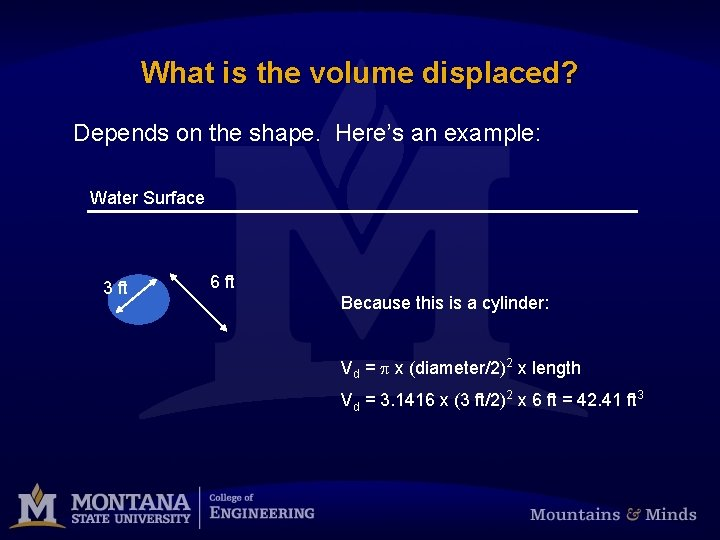What is the volume displaced? Depends on the shape. Here’s an example: Water Surface 3 ft 6 ft Because this is a cylinder: Vd = p x (diameter/2)2 x length Vd = 3. 1416 x (3 ft/2)2 x 6 ft = 42. 41 ft 3Now what, does it float or not? Let’s say that an object is submerged in the water and then released. At the moment it is released, there is nothing holding it up from above and it is not resting on a solid object below. That’s the same as saying the supporting force, Fe, is zero. Fe = 0 w Fb = w Fb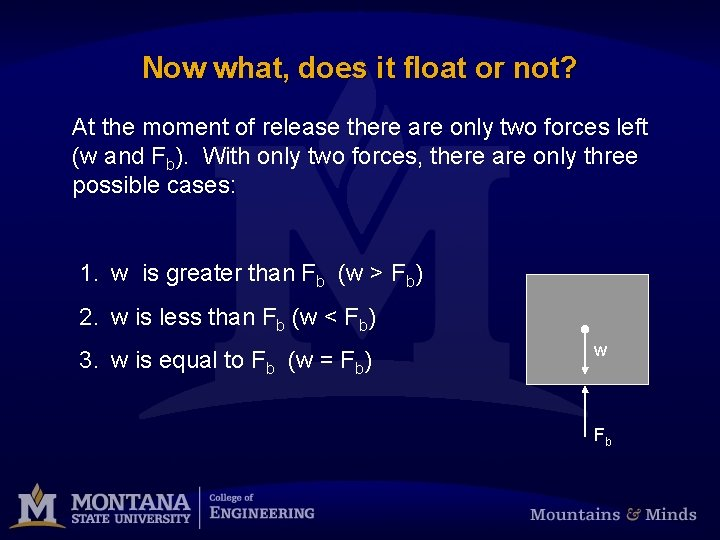Now what, does it float or not? At the moment of release there are only two forces left (w and Fb). With only two forces, there are only three possible cases: 1. w is greater than Fb (w > Fb) 2. w is less than Fb (w < Fb) 3. w is equal to Fb (w = Fb) w FbCase 1 1. w is greater than Fb (w > Fb) If w is greater than Fb, then the sum of the forces is (w points downward, so w is negative): sum of forces = (-w) + Fb which will be a negative number! Side Note: Notice the length of the arrows.Case 1 1. w is greater than Fb (w > Fb) w If the sum of forces is non-zero, then the object is accelerating. Since the sum of forces is negative, the object is accelerating downward. The object is sinking! Fb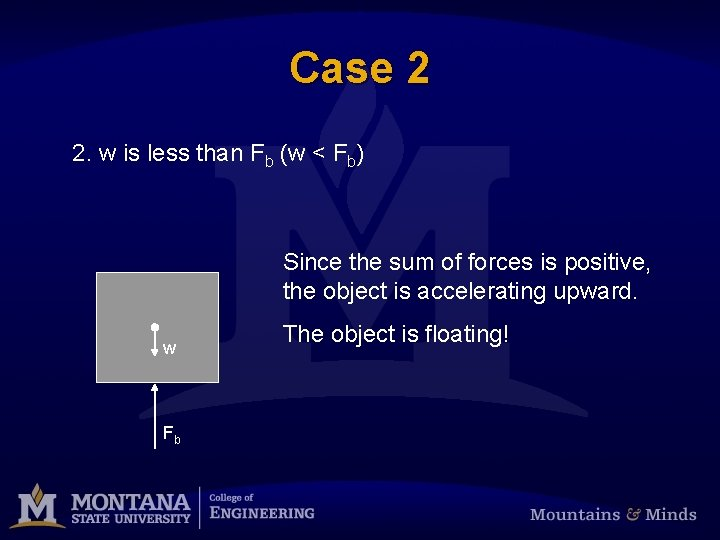Case 2 2. w is less than Fb (w < Fb) Since the sum of forces is positive, the object is accelerating upward. w Fb The object is floating!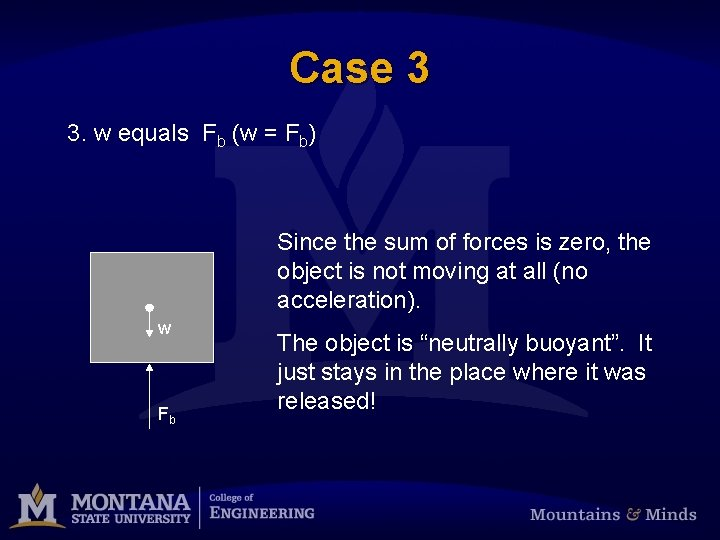Case 3 3. w equals Fb (w = Fb) Since the sum of forces is zero, the object is not moving at all (no acceleration). w Fb The object is “neutrally buoyant”. It just stays in the place where it was released!An example A brick is 6 inches long, 3 inches wide and 3 inches tall. The brick was placed on a scale and weighed 4 lb. When submerged and then released, will the brick float? 6 in 3 in The weight of the object, w, is 4 lb. w = 4 lb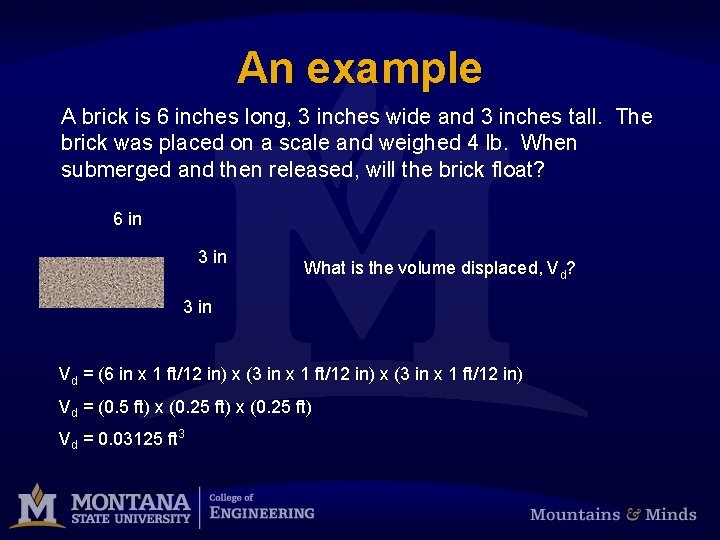An example A brick is 6 inches long, 3 inches wide and 3 inches tall. The brick was placed on a scale and weighed 4 lb. When submerged and then released, will the brick float? 6 in 3 in What is the volume displaced, Vd? 3 in Vd = (6 in x 1 ft/12 in) x (3 in x 1 ft/12 in) Vd = (0. 5 ft) x (0. 25 ft) Vd = 0. 03125 ft 3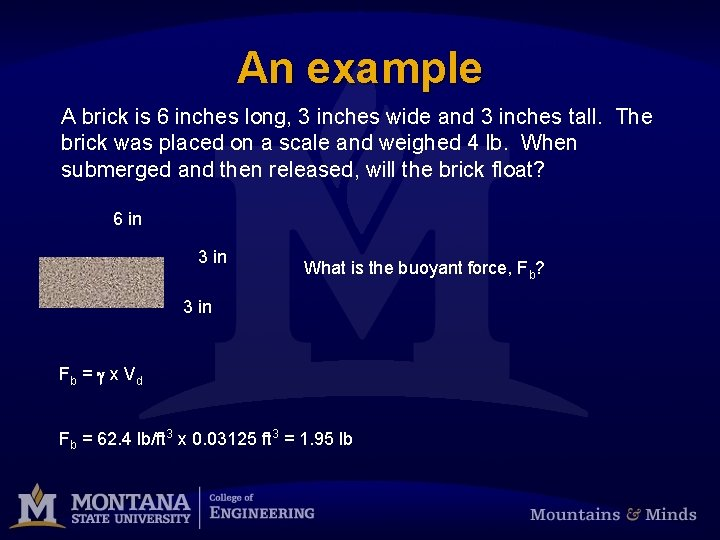An example A brick is 6 inches long, 3 inches wide and 3 inches tall. The brick was placed on a scale and weighed 4 lb. When submerged and then released, will the brick float? 6 in 3 in What is the buoyant force, Fb? 3 in Fb = g x V d Fb = 62. 4 lb/ft 3 x 0. 03125 ft 3 = 1. 95 lb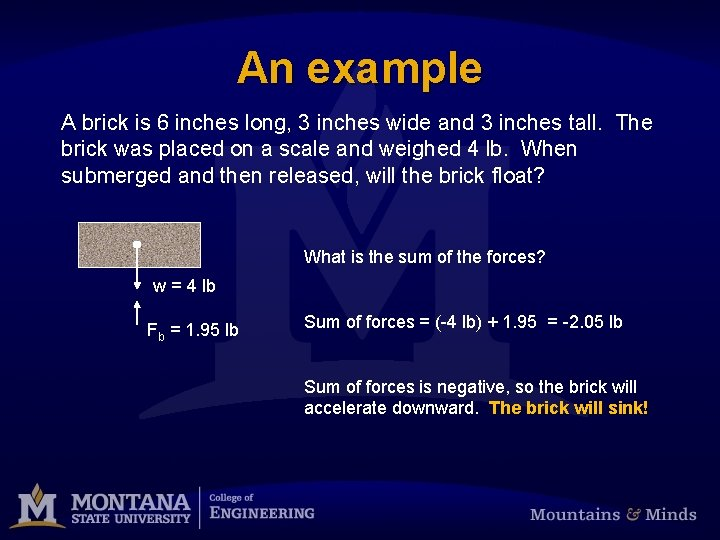An example A brick is 6 inches long, 3 inches wide and 3 inches tall. The brick was placed on a scale and weighed 4 lb. When submerged and then released, will the brick float? What is the sum of the forces? w = 4 lb Fb = 1. 95 lb Sum of forces = (-4 lb) + 1. 95 = -2. 05 lb Sum of forces is negative, so the brick will accelerate downward. The brick will sink!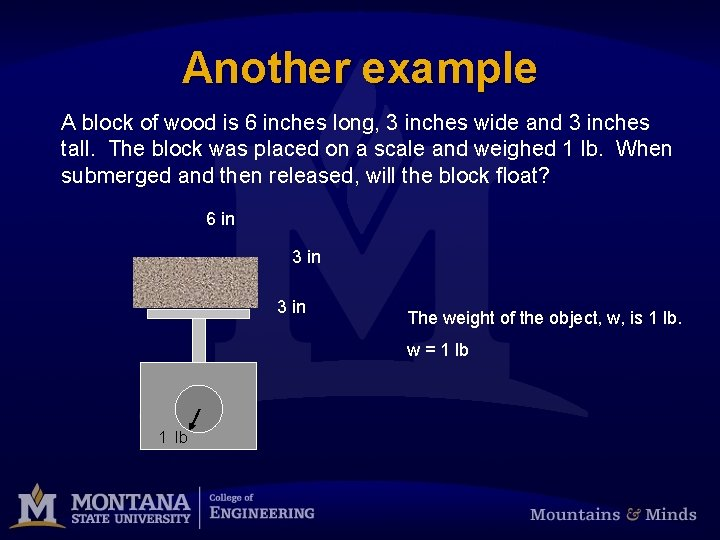Another example A block of wood is 6 inches long, 3 inches wide and 3 inches tall. The block was placed on a scale and weighed 1 lb. When submerged and then released, will the block float? 6 in 3 in The weight of the object, w, is 1 lb. w = 1 lb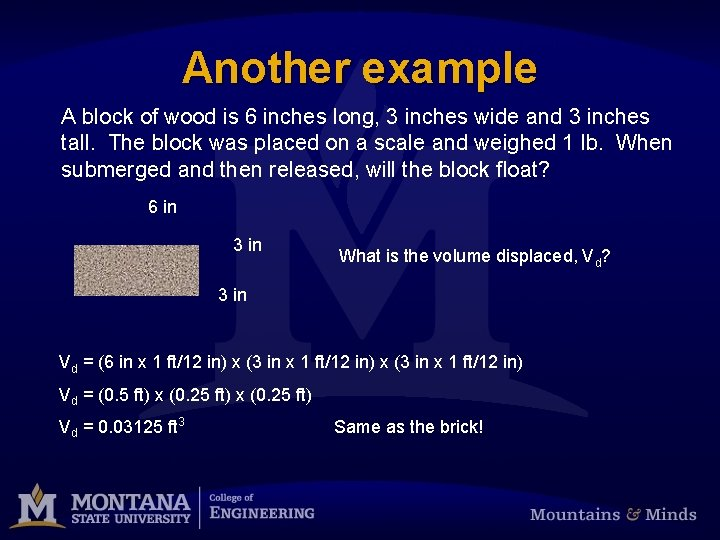Another example A block of wood is 6 inches long, 3 inches wide and 3 inches tall. The block was placed on a scale and weighed 1 lb. When submerged and then released, will the block float? 6 in 3 in What is the volume displaced, Vd? 3 in Vd = (6 in x 1 ft/12 in) x (3 in x 1 ft/12 in) Vd = (0. 5 ft) x (0. 25 ft) Vd = 0. 03125 ft 3 Same as the brick!Another example A block of wood is 6 inches long, 3 inches wide and 3 inches tall. The block was placed on a scale and weighed 1 lb. When submerged and then released, will the block float? 6 in 3 in What is the buoyant force, Fb? 3 in Fb = g x V d Fb = 62. 4 lb/ft 3 x 0. 03125 ft 3 = 1. 95 lb Same as the brick!Another example A block of wood is 6 inches long, 3 inches wide and 3 inches tall. The block was placed on a scale and weighed 1 lb. When submerged and then released, will the block float? What is the sum of the forces? w = 4 lb Fb = 1. 95 lb Sum of forces = (-1 lb) + 1. 95 = 0. 95 lb Sum of forces is positive, so the brick will accelerate upward. The wood block will float!A third example A block of wood is 6 inches long, 3 inches wide and 3 inches tall. The block was placed on a scale and weighed 1 lb. When submerged and then released, we have shown the block will float. Now, how much of the block will be above water? Or like this? Water Surface Like this?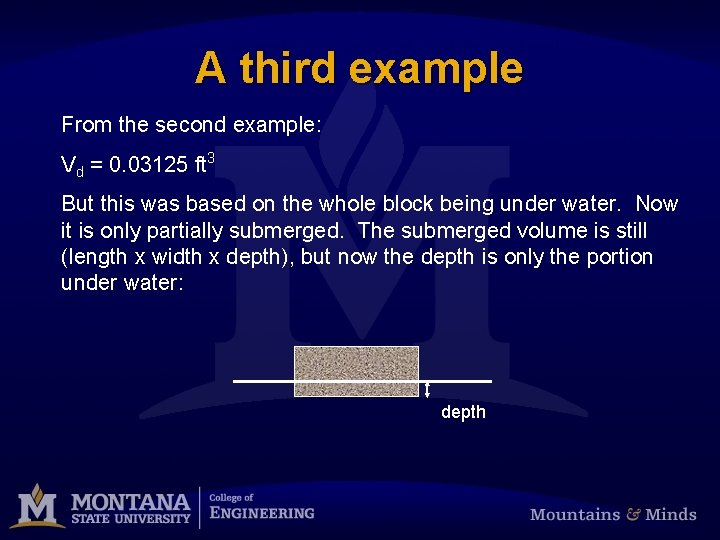A third example From the second example: Vd = 0. 03125 ft 3 But this was based on the whole block being under water. Now it is only partially submerged. The submerged volume is still (length x width x depth), but now the depth is only the portion under water: depth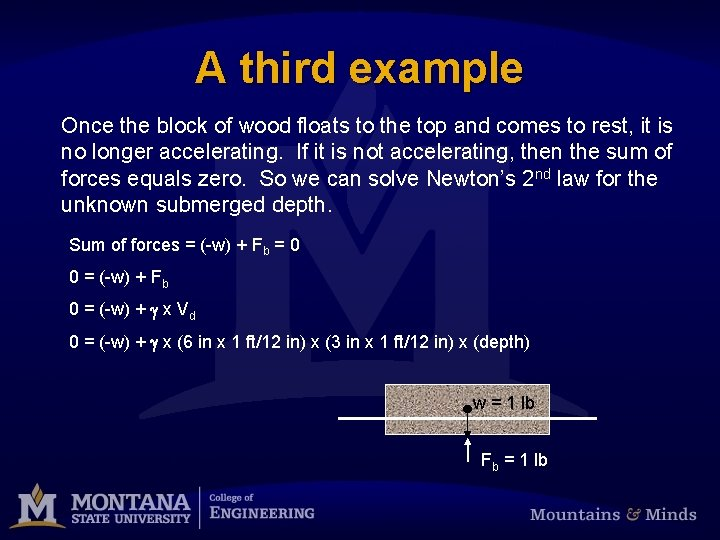A third example Once the block of wood floats to the top and comes to rest, it is no longer accelerating. If it is not accelerating, then the sum of forces equals zero. So we can solve Newton’s 2 nd law for the unknown submerged depth. Sum of forces = (-w) + Fb = 0 0 = (-w) + Fb 0 = (-w) + g x Vd 0 = (-w) + g x (6 in x 1 ft/12 in) x (3 in x 1 ft/12 in) x (depth) w = 1 lb Fb = 1 lb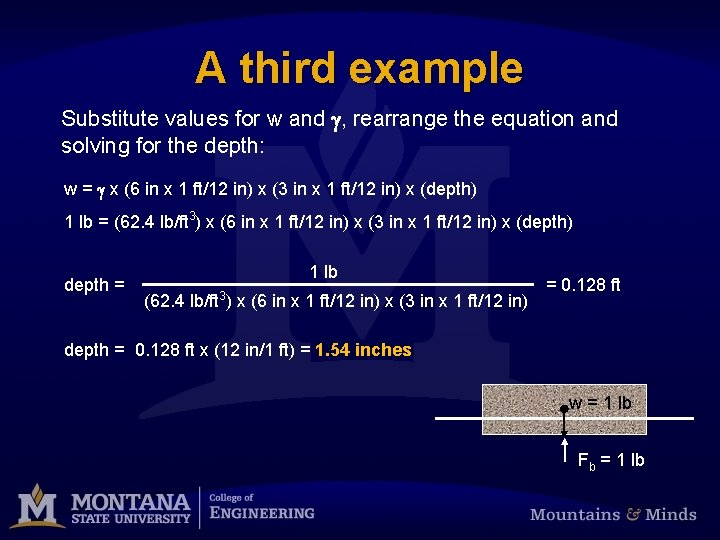A third example Substitute values for w and g, rearrange the equation and solving for the depth: w = g x (6 in x 1 ft/12 in) x (3 in x 1 ft/12 in) x (depth) 1 lb = (62. 4 lb/ft 3) x (6 in x 1 ft/12 in) x (3 in x 1 ft/12 in) x (depth) depth = 1 lb 3 (62. 4 lb/ft ) x (6 in x 1 ft/12 in) x (3 in x 1 ft/12 in) = 0. 128 ft depth = 0. 128 ft x (12 in/1 ft) = 1. 54 inches w = 1 lb Fb = 1 lb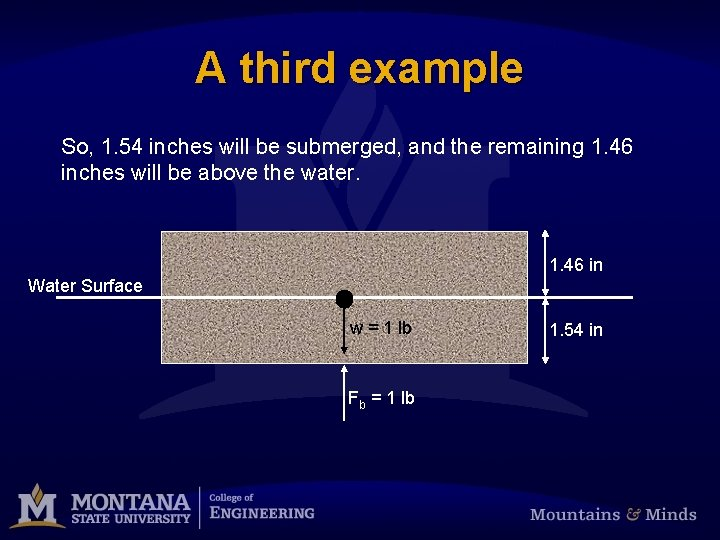A third example So, 1. 54 inches will be submerged, and the remaining 1. 46 inches will be above the water. 1. 46 in Water Surface w = 1 lb Fb = 1 lb 1. 54 in